Related Articles

# Residual Networks (ResNet) – Deep Learning

• Last Updated : 03 Jun, 2020

After the first CNN-based architecture (AlexNet) that win the ImageNet 2012 competition, Every subsequent winning architecture uses more layers in a deep neural network to reduce the error rate. This works for less number of layers, but when we increase the number of layers, there is a common problem in deep learning associated with that called Vanishing/Exploding gradient. This causes the gradient to become 0 or too large. Thus when we increases number of layers, the training and test error rate also increases.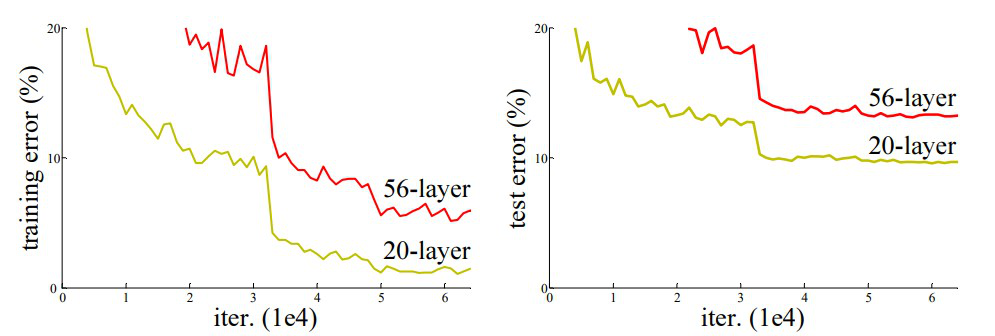In the above plot, we can observe that a 56-layer CNN gives more error rate on both training and testing dataset than a 20-layer CNN architecture, If this was the result of over fitting, then we should have lower training error in 56-layer CNN but then it also has higher training error. After analyzing more on error rate the authors were able to reach conclusion that it is caused by vanishing/exploding gradient.

ResNet, which was proposed in 2015 by researchers at Microsoft Research introduced a new architecture called Residual Network.

Residual Block:
In order to solve the problem of the vanishing/exploding gradient, this architecture introduced the concept called Residual Network. In this network we use a technique called skip connections . The skip connection skips training from a few layers and connects directly to the output.

The approach behind this network is instead of layers learn the underlying mapping, we allow network fit the residual mapping. So, instead of say H(x), initial mapping, let the network fit, F(x) := H(x) – x which gives H(x) := F(x) + x.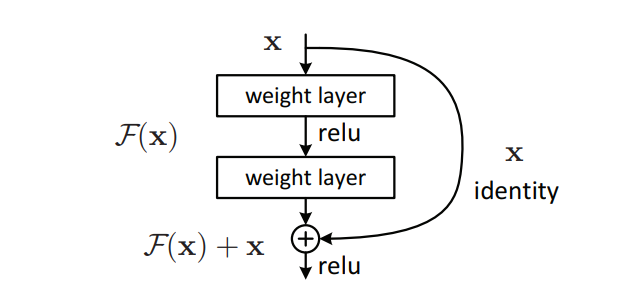The advantage of adding this type of skip connection is because if any layer hurt the performance of architecture then it will be skipped by regularization. So, this results in training very deep neural network without the problems caused by vanishing/exploding gradient.  The authors of the paper experimented on 100-1000 layers on CIFAR-10 dataset.

There is a similar approach called “highway networks”, these networks also uses skip connection. Similar to LSTM these skip connections also uses parametric gates. These gates determine how much information passes through the skip connection. This architecture however  has not provide accuracy better than ResNet architecture.

Network Architecture:

This network uses a 34-layer plain network architecture inspired by VGG-19 in which then the shortcut connection is added. These shortcut connections then convert the architecture into residual network.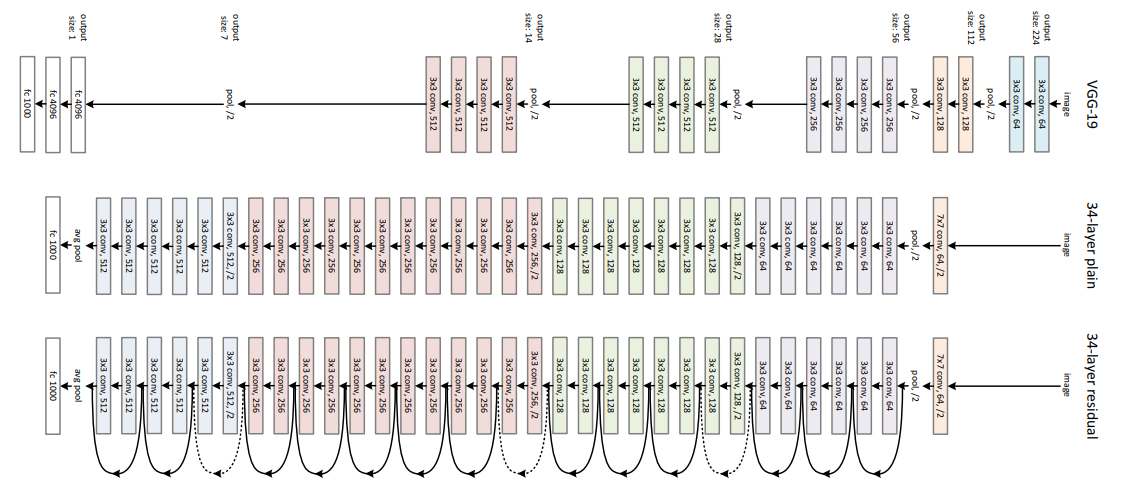Implementation:

Using the Tensorflow and Keras API, we can design ResNet architecture (including Residual Blocks) from scratch. Below is the implementation of different ResNet architecture. For this implementation we use CIFAR-10 dataset. This dataset contains 60, 000 32×32 color images in 10 different classes (airplanes, cars, birds, cats, deer, dogs, frogs, horses, ships, and trucks) etc. This datasets can be assessed  from keras.datasets API function.

• First, we import the keras module and its APIs. These APIs help in building architecture of the ResNet model.
Code: Importing Libraries
 `# Import Keras modules and its important APIs``import` `keras``from` `keras.layers ``import` `Dense, Conv2D, BatchNormalization, Activation``from` `keras.layers ``import` `AveragePooling2D, ``Input``, Flatten``from` `keras.optimizers ``import` `Adam``from` `keras.callbacks ``import` `ModelCheckpoint, LearningRateScheduler``from` `keras.callbacks ``import` `ReduceLROnPlateau``from` `keras.preprocessing.image ``import` `ImageDataGenerator``from` `keras.regularizers ``import` `l2``from` `keras ``import` `backend as K``from` `keras.models ``import` `Model``from` `keras.datasets ``import` `cifar10``import` `numpy as np``import` `os`
• Now, We set different hyper parameters that is required for ResNet architecture. We also done some preprocess our datasets to prepare it for training.
Code: Setting Training Hyperparameters
 `# Setting Training Hyperparameters``batch_size ``=` `32`  `# original ResNet paper uses batch_size = 128 for training``epochs ``=` `200``data_augmentation ``=` `True``num_classes ``=` `10`` ` `# Data Preprocessing ``subtract_pixel_mean ``=` `True``n ``=` `3`` ` `# Select ResNet Version``version ``=` `1`` ` `# Computed depth of ``if` `version ``=``=` `1``:``    ``depth ``=` `n ``*` `6` `+` `2``elif` `version ``=``=` `2``:``    ``depth ``=` `n ``*` `9` `+` `2`` ` `# Model name, depth and version``model_type ``=` `'ResNet % dv % d'` `%` `(depth, version)`` ` `# Load the CIFAR-10 data.``(x_train, y_train), (x_test, y_test) ``=` `cifar10.load_data()`` ` `# Input image dimensions.``input_shape ``=` `x_train.shape[``1``:]`` ` `# Normalize data.``x_train ``=` `x_train.astype(``'float32'``) ``/` `255``x_test ``=` `x_test.astype(``'float32'``) ``/` `255`` ` `# If subtract pixel mean is enabled``if` `subtract_pixel_mean:``    ``x_train_mean ``=` `np.mean(x_train, axis ``=` `0``)``    ``x_train ``-``=` `x_train_mean``    ``x_test ``-``=` `x_train_mean`` ` `# Print Training and Test Samples ``print``(``'x_train shape:'``, x_train.shape)``print``(x_train.shape[``0``], ``'train samples'``)``print``(x_test.shape[``0``], ``'test samples'``)``print``(``'y_train shape:'``, y_train.shape)`` ` `# Convert class vectors to binary class matrices.``y_train ``=` `keras.utils.to_categorical(y_train, num_classes)``y_test ``=` `keras.utils.to_categorical(y_test, num_classes)`
• In this step, we set the learning rate according to the number of epochs. As the number of epochs the learning rate must be decreased to ensure better learning.

Code: Setting LR for different number of Epochs

 `# Setting LR for different number of Epochs``def` `lr_schedule(epoch):``    ``lr ``=` `1e``-``3``    ``if` `epoch > ``180``:``        ``lr ``*``=` `0.5e``-``3``    ``elif` `epoch > ``160``:``        ``lr ``*``=` `1e``-``3``    ``elif` `epoch > ``120``:``        ``lr ``*``=` `1e``-``2``    ``elif` `epoch > ``80``:``        ``lr ``*``=` `1e``-``1``    ``print``(``'Learning rate: '``, lr)``    ``return` `lr`
• In this step we define basic ResNet building block that can be used for defining the ResNet V1 and V2 architecture.
Code: Basic ResNet Building Block
 `# Basic ResNet Building Block``def` `resnet_layer(inputs,``                 ``num_filters ``=` `16``,``                 ``kernel_size ``=` `3``,``                 ``strides ``=` `1``,``                 ``activation ``=``'relu'``,``                 ``batch_normalization ``=` `True``,``    ``conv ``=` `Conv2D(num_filters,``                  ``kernel_size ``=` `kernel_size,``                  ``strides ``=` `strides,``                  ``padding ``=``'same'``,``                  ``kernel_initializer ``=``'he_normal'``,``                  ``kernel_regularizer ``=` `l2(``1e``-``4``))`` ` `    ``x ``=` `inputs``    ``if` `conv_first:``        ``x ``=` `conv(x)``        ``if` `batch_normalization:``            ``x ``=` `BatchNormalization()(x)``        ``if` `activation ``is` `not` `None``:``            ``x ``=` `Activation(activation)(x)``    ``else``:``        ``if` `batch_normalization:``            ``x ``=` `BatchNormalization()(x)``        ``if` `activation ``is` `not` `None``:``            ``x ``=` `Activation(activation)(x)``        ``x ``=` `conv(x)``    ``return` `x`
• In this step we define ResNet V1 architecture that is based on the ResNet building block we defined above:
Code: ResNet V1 architecture

 `# ``def` `resnet_v1(input_shape, depth, num_classes ``=` `10``):``     ` `    ``if` `(depth ``-` `2``) ``%` `6` `!``=` `0``:``        ``raise` `ValueError(``'depth should be 6n + 2 (eg 20, 32, 44 in [a])'``)``    ``# Start model definition.``    ``num_filters ``=` `16``    ``num_res_blocks ``=` `int``((depth ``-` `2``) ``/` `6``)`` ` `    ``inputs ``=` `Input``(shape ``=` `input_shape)``    ``x ``=` `resnet_layer(inputs ``=` `inputs)``    ``# Instantiate the stack of residual units``    ``for` `stack ``in` `range``(``3``):``        ``for` `res_block ``in` `range``(num_res_blocks):``            ``strides ``=` `1``            ``if` `stack > ``0` `and` `res_block ``=``=` `0``:  ``# first layer but not first stack``                ``strides ``=` `2`  `# downsample``            ``y ``=` `resnet_layer(inputs ``=` `x,``                             ``num_filters ``=` `num_filters,``                             ``strides ``=` `strides)``            ``y ``=` `resnet_layer(inputs ``=` `y,``                             ``num_filters ``=` `num_filters,``                             ``activation ``=` `None``)``            ``if` `stack > ``0` `and` `res_block ``=``=` `0``:  ``# first layer but not first stack``                ``# linear projection residual shortcut connection to match``                ``# changed dims``                ``x ``=` `resnet_layer(inputs ``=` `x,``                                 ``num_filters ``=` `num_filters,``                                 ``kernel_size ``=` `1``,``                                 ``strides ``=` `strides,``                                 ``activation ``=` `None``,``                                 ``batch_normalization ``=` `False``)``            ``x ``=` `keras.layers.add([x, y])``            ``x ``=` `Activation(``'relu'``)(x)``        ``num_filters ``*``=` `2`` ` `    ``# Add classifier on top.``    ``# v1 does not use BN after last shortcut connection-ReLU``    ``x ``=` `AveragePooling2D(pool_size ``=` `8``)(x)``    ``y ``=` `Flatten()(x)``    ``outputs ``=` `Dense(num_classes,``                    ``activation ``=``'softmax'``,``                    ``kernel_initializer ``=``'he_normal'``)(y)`` ` `    ``# Instantiate model.``    ``model ``=` `Model(inputs ``=` `inputs, outputs ``=` `outputs)``    ``return` `model`
• In this step we define ResNet V2 architecture that is based on the ResNet building block we defined above:
Code: ResNet V2 architecture
 `# ResNet V2 architecture``def` `resnet_v2(input_shape, depth, num_classes ``=` `10``):``    ``if` `(depth ``-` `2``) ``%` `9` `!``=` `0``:``        ``raise` `ValueError(``'depth should be 9n + 2 (eg 56 or 110 in [b])'``)``    ``# Start model definition.``    ``num_filters_in ``=` `16``    ``num_res_blocks ``=` `int``((depth ``-` `2``) ``/` `9``)`` ` `    ``inputs ``=` `Input``(shape ``=` `input_shape)``    ``# v2 performs Conv2D with BN-ReLU on input before splitting into 2 paths``    ``x ``=` `resnet_layer(inputs ``=` `inputs,``                     ``num_filters ``=` `num_filters_in,``                     ``conv_first ``=` `True``)`` ` `    ``# Instantiate the stack of residual units``    ``for` `stage ``in` `range``(``3``):``        ``for` `res_block ``in` `range``(num_res_blocks):``            ``activation ``=` `'relu'``            ``batch_normalization ``=` `True``            ``strides ``=` `1``            ``if` `stage ``=``=` `0``:``                ``num_filters_out ``=` `num_filters_in ``*` `4``                ``if` `res_block ``=``=` `0``:  ``# first layer and first stage``                    ``activation ``=` `None``                    ``batch_normalization ``=` `False``            ``else``:``                ``num_filters_out ``=` `num_filters_in ``*` `2``                ``if` `res_block ``=``=` `0``:  ``# first layer but not first stage``                    ``strides ``=` `2`    `# downsample`` ` `            ``# bottleneck residual unit``            ``y ``=` `resnet_layer(inputs ``=` `x,``                             ``num_filters ``=` `num_filters_in,``                             ``kernel_size ``=` `1``,``                             ``strides ``=` `strides,``                             ``activation ``=` `activation,``                             ``batch_normalization ``=` `batch_normalization,``                             ``conv_first ``=` `False``)``            ``y ``=` `resnet_layer(inputs ``=` `y,``                             ``num_filters ``=` `num_filters_in,``                             ``conv_first ``=` `False``)``            ``y ``=` `resnet_layer(inputs ``=` `y,``                             ``num_filters ``=` `num_filters_out,``                             ``kernel_size ``=` `1``,``                             ``conv_first ``=` `False``)``            ``if` `res_block ``=``=` `0``:``                ``# linear projection residual shortcut connection to match``                ``# changed dims``                ``x ``=` `resnet_layer(inputs ``=` `x,``                                 ``num_filters ``=` `num_filters_out,``                                 ``kernel_size ``=` `1``,``                                 ``strides ``=` `strides,``                                 ``activation ``=` `None``,``                                 ``batch_normalization ``=` `False``)``            ``x ``=` `keras.layers.add([x, y])`` ` `        ``num_filters_in ``=` `num_filters_out`` ` `    ``# Add classifier on top.``    ``# v2 has BN-ReLU before Pooling``    ``x ``=` `BatchNormalization()(x)``    ``x ``=` `Activation(``'relu'``)(x)``    ``x ``=` `AveragePooling2D(pool_size ``=` `8``)(x)``    ``y ``=` `Flatten()(x)``    ``outputs ``=` `Dense(num_classes,``                    ``activation ``=``'softmax'``,``                    ``kernel_initializer ``=``'he_normal'``)(y)`` ` `    ``# Instantiate model.``    ``model ``=` `Model(inputs ``=` `inputs, outputs ``=` `outputs)``    ``return` `model`
• The code below is used to train and test the ResNet v1 and v2 architecture we defined above:
Code: Main function
 `# Main function ``if` `version ``=``=` `2``:``    ``model ``=` `resnet_v2(input_shape ``=` `input_shape, depth ``=` `depth)``else``:``    ``model ``=` `resnet_v1(input_shape ``=` `input_shape, depth ``=` `depth)`` ` `model.``compile``(loss ``=``'categorical_crossentropy'``,``              ``optimizer ``=` `Adam(learning_rate ``=` `lr_schedule(``0``)),``              ``metrics ``=``[``'accuracy'``])``model.summary()``print``(model_type)`` ` `# Prepare model model saving directory.``save_dir ``=` `os.path.join(os.getcwd(), ``'saved_models'``)``model_name ``=` `'cifar10_% s_model.{epoch:03d}.h5'` `%` `model_type``if` `not` `os.path.isdir(save_dir):``    ``os.makedirs(save_dir)``filepath ``=` `os.path.join(save_dir, model_name)`` ` `# Prepare callbacks for model saving and for learning rate adjustment.``checkpoint ``=` `ModelCheckpoint(filepath ``=` `filepath,``                             ``monitor ``=``'val_acc'``,``                             ``verbose ``=` `1``,``                             ``save_best_only ``=` `True``)`` ` `lr_scheduler ``=` `LearningRateScheduler(lr_schedule)`` ` `lr_reducer ``=` `ReduceLROnPlateau(factor ``=` `np.sqrt(``0.1``),``                               ``cooldown ``=` `0``,``                               ``patience ``=` `5``,``                               ``min_lr ``=` `0.5e``-``6``)`` ` `callbacks ``=` `[checkpoint, lr_reducer, lr_scheduler]`` ` `# Run training, with or without data augmentation.``if` `not` `data_augmentation:``    ``print``(``'Not using data augmentation.'``)``    ``model.fit(x_train, y_train,``              ``batch_size ``=` `batch_size,``              ``epochs ``=` `epochs,``              ``validation_data ``=``(x_test, y_test),``              ``shuffle ``=` `True``,``              ``callbacks ``=` `callbacks)``else``:``    ``print``(``'Using real-time data augmentation.'``)``    ``# This will do preprocessing and realtime data augmentation:``    ``datagen ``=` `ImageDataGenerator(``        ``# set input mean to 0 over the dataset``        ``featurewise_center ``=` `False``,``        ``# set each sample mean to 0``        ``samplewise_center ``=` `False``,``        ``# divide inputs by std of dataset``        ``featurewise_std_normalization ``=` `False``,``        ``# divide each input by its std``        ``samplewise_std_normalization ``=` `False``,``        ``# apply ZCA whitening``        ``zca_whitening ``=` `False``,``        ``# epsilon for ZCA whitening``        ``zca_epsilon ``=` `1e``-``06``,``        ``# randomly rotate images in the range (deg 0 to 180)``        ``rotation_range ``=` `0``,``        ``# randomly shift images horizontally``        ``width_shift_range ``=` `0.1``,``        ``# randomly shift images vertically``        ``height_shift_range ``=` `0.1``,``        ``# set range for random shear``        ``shear_range ``=` `0.``,``        ``# set range for random zoom``        ``zoom_range ``=` `0.``,``        ``# set range for random channel shifts``        ``channel_shift_range ``=` `0.``,``        ``# set mode for filling points outside the input boundaries``        ``fill_mode ``=``'nearest'``,``        ``# value used for fill_mode = "constant"``        ``cval ``=` `0.``,``        ``# randomly flip images``        ``horizontal_flip ``=` `True``,``        ``# randomly flip images``        ``vertical_flip ``=` `False``,``        ``# set rescaling factor (applied before any other transformation)``        ``rescale ``=` `None``,``        ``# set function that will be applied on each input``        ``preprocessing_function ``=` `None``,``        ``# image data format, either "channels_first" or "channels_last"``        ``data_format ``=` `None``,``        ``# fraction of images reserved for validation (strictly between 0 and 1)``        ``validation_split ``=` `0.0``)`` ` `    ``# Compute quantities required for featurewise normalization``    ``# (std, mean, and principal components if ZCA whitening is applied).``    ``datagen.fit(x_train)`` ` `    ``# Fit the model on the batches generated by datagen.flow().``    ``model.fit_generator(datagen.flow(x_train, y_train, batch_size ``=` `batch_size),``                        ``validation_data ``=``(x_test, y_test),``                        ``epochs ``=` `epochs, verbose ``=` `1``, workers ``=` `4``,``                        ``callbacks ``=` `callbacks)`` ` `# Score trained model.``scores ``=` `model.evaluate(x_test, y_test, verbose ``=` `1``)``print``(``'Test loss:'``, scores[``0``])``print``(``'Test accuracy:'``, scores[``1``])`

Results & Conclusion:

On the ImageNet dataset,  the authors uses a 152-layers ResNet, which is 8 times more deep than VGG19 but still have less parameters. An ensemble of these ResNets generated an error of only 3.7% on ImageNet test set, the result which won ILSVRC 2015 competition. On COCO object detection dataset, it also generates a 28% relative improvement due to its very deep representation.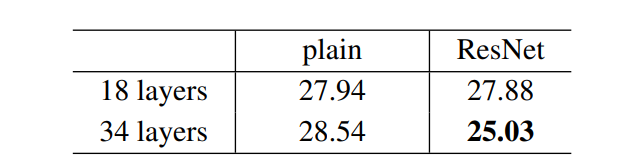• The result above shows that shortcut connections would be able to solve the problem caused by increasing the layers because as we increase layers from 18 to 34 the error rate on ImageNet Validation Set also decreases unlike the plain network.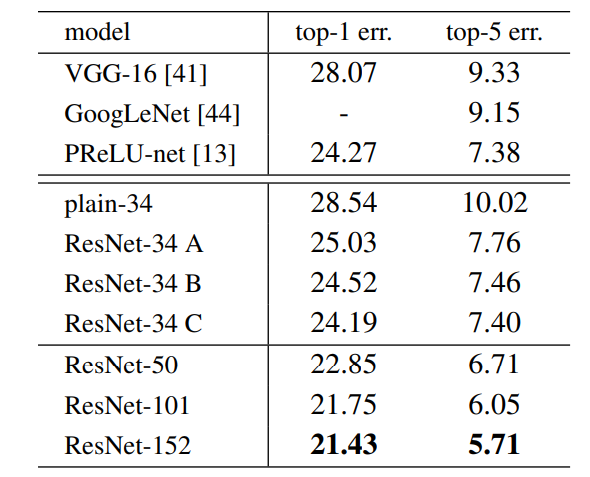• Below are the results on ImageNet Test Set. The 3.57% top-5 error rate of ResNet was the lowest and thus ResNet architecture came first in ImageNet classification challenge in 2015.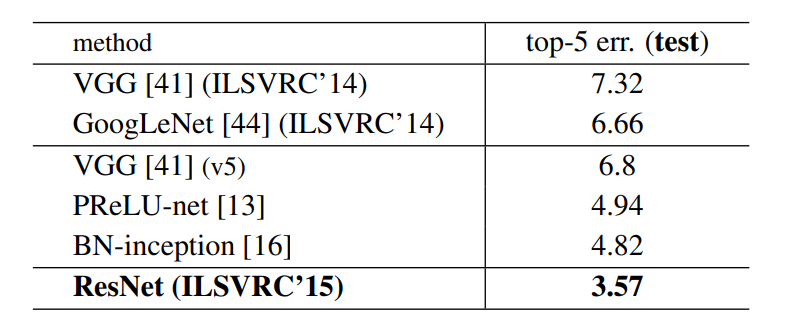References:

Attention reader! Don’t stop learning now. Get hold of all the important Machine Learning Concepts with the Machine Learning Foundation Course at a student-friendly price and become industry ready.

My Personal Notes arrow_drop_up